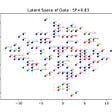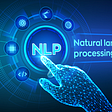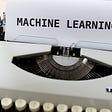# Top 6 Machine Learning Algorithms

In this blog, I will talk about famous machine learning algorithms used for building machine learning models. You’ll find usage of these algorithms whenever you’re trying to extract information from data, write code to predict things or trying to find inference from data.

The aim here is to provide a one-stop revision of basic ML algorithms for interviews and exam preparation. So, keep reading the blog to find more about famous ML algorithms.

# 1. Linear Regression

Linear regression is a predictive statistical approach for modelling relationship between a dependent variable with a given set of independent variables.

It is a linear approach to modeling the relationship between a dependent variable and one or more independent variables. When we have only one independent variable it is as called simple linear regression. For more than one independent variable, the process is called multiple linear regression.

# 2. Logistic Regression

Logistic Regression is a Supervised learning algorithm widely used for classification. It is used to predict a binary outcome (1/ 0, Yes/ No, True/ False) given a set of independent variables. To represent binary/ categorical outcome, we use dummy variables.

Logistic regression uses an equation as the representation, very much like linear regression. It is not much different from linear regression, except that a Sigmoid function is being fit in the equation of linear regression.

## Advantages of Linear Regression :

1. It makes no assumptions about distributions of classes in feature space.
2. Easily extended to multiple classes (multinomial regression).
3. Natural probabilistic view of class predictions.
4. Quick to train and very fast at classifying unknown records.
5. Good accuracy for many simple data sets.
6. Resistant to overfitting.

## Disadvantages of Logistic Regression :

1. It cannot handle continuous variables.
2. If independent variables are not correlated with the target variable, then Logistic regression does not work.
3. Requires large sample size for stable results.

# 2. Support Vector Machines (SVM)

Support vector machines (SVMs) are a set of supervised learning methods used for classification, regression and outlier detection.

1. They maximize the margin of decision boundary
2. They can handle large feature spaces.
3. SVMs work well with semi-structured and unstructured data.
4. They can use the concept of kernel trick to solve any complex problem.

1. SVMs can be difficult to implement when the number of classes is more than 2.
2. SVMs take a long time for training and they are sensitive to noise.
3. Choosing a good kernel function is not easy in SVM and requires a lot of testing.
4. Hyperparameters like gamma and cost-C are not easy to fine-tune.

# 3. K-Nearest Neighbors (KNN)

K- Nearest Neighbors is a Supervised machine learning algorithm which can be used for classification as well as regression. It does not make an assumption about the underlying data distribution pattern.

The classification of objects in KNN is made on the basis of plurality of vote of its closest K neighbors, where K can be any small positive integer. The algorithm eagerly learns during the training step. It can predict whether a new point will fall into a given cluster.

## Steps to apply K-Nearest Neighbors Algorithms:

1. Select a value for K (odd number)
2. Choose a sample data point that needs to be classified and compute distance to its n training samples.
3. Sort the distances and take K closest samples.
4. Assign the sample data point to the class that has the majority vote of its K neighbors.

## Cons of KNN Algorithms:

KNN is computationally expensive as it searches the nearest neighbors for the new point at the prediction stage. Memory requirement for KNN is high. Sensitive to outliers, accuracy is impacted by noise or irrelevant data.

# 4. XGBoost (eXtreme Gradient Boosting)

Extreme Gradient Boosting (XGBoost) is an open-source library that provides an efficient and effective implementation of the gradient boosting algorithm.

Gradient boosting belongs to ensemble machine learning algorithms that can be used for classification or regression predictive modeling problems.

In an Ensemble method the algorithm combines the predictions from multiple machine learning algorithms together to make more accurate predictions than any individual model.

## Some of the reasons why XGBoost is used:

1. It is a powerful algorithm with high speed and performance.
2. Processing power of modern multicore computers can be utilized by the XGBoost algorithm.
3. It is cost-effective to train on large datasets.
4. Consistently outperform all single algorithm methods.

AdaBoost is a Boosting Algorithm which includes a group of algorithms that utilize weighted averages to make weak learners into strong learners. Each model that runs decides what features the next model will focus on.

The performance of decision trees on binary classification problems is frequently boosted using the AdaBoost algorithm. It is done by building a model from the training data, then creating a second model that attempts to correct the errors from the first model.

AdaBoost keeps on adding the models until the training set starts getting predicted perfectly or a given number of models is added. It is less susceptible to over-fitting than other learning algorithms.

# 6. Artificial Neural Network ( ANN )

ANNs are composed of multiple nodes, which imitate biological neurons of the human brain. The node is connected via links and they can interact with each other.

Each node takes input data and performs simple operations on that data. The results are passed on to other nodes. The output from each node is called its activation. Each connection from a node has a weight associated with it. ANNs learn by altering weight values each time.

The list itself is not exhaustive and we have certainly not discussed popular tree-based algorithms like Decision Trees and Random Forest algorithms. For more information about them, refer the links above.

There you go, you have learned about the Top 6 Machine Learning Algorithms that you can apply on datasets and build machine learning models. Make sure to keep these algorithms in mind the next time you are working on a Machine Learning project.

--

--

--

## More from Afroz Chakure

300k+ Views on Medium | 4xTop Writer | Technology, Productivity, Books and Life | Linkedin: linkedin.com/in/afroz-chakure-489780168 | InteractiveGeneration.tech

Love podcasts or audiobooks? Learn on the go with our new app.

## State of the art NLP at scale with RAPIDS, HuggingFace and Dask## Course Review: CS 7642 Reinforcement Learning — OMSCS Georgia Institute of Technology## Catalyst.Neuro: A 3D Brain Segmentation Pipeline for MRI## Learning from unlabelled data with COVID-19 Open Research Dataset## Multinomial Mixture Model for Supermarket Shoppers Segmentation (A complete tutorial)## Dynamic Self Organizing Maps (GSOM)## NLP In TensorFlow Using Transfer Learning.## Afroz Chakure

300k+ Views on Medium | 4xTop Writer | Technology, Productivity, Books and Life | Linkedin: linkedin.com/in/afroz-chakure-489780168 | InteractiveGeneration.tech

## 6 Steps to Become a Machine Learning Expert## Complete roadmap to be a deep learning engineer## 7 Steps to build a Machine Learning Model## All you need to know about Overfitting and Underfitting in building Machine Learning Models.